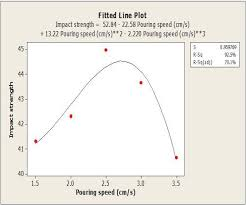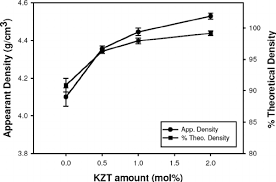## How to Calculate and Solve for Pouring Speed | Design of Gating SystemThe image above represents pouring speed.

To compute for poring speed, three essential parameters are needed and these parameters are Co-efficient for friction (μ), Acceleration due to Gravity (g) and Rated head static (Hs).

The formula for calculating pouring speed:

v = μ√(2gHs)

Where:

v = Pouring Speed
μ = Co-efficient for Friction
g = Acceleration due to Gravity

Let’s solve an example;
Find the pouring speed when the co-efficient for friction is 14, acceleration due to gravity is 9 and rated static head is 16.

This implies that;

μ = Co-efficient for Friction = 14
g = Acceleration due to Gravity = 9
Hs = Rated Static Head = 16

v = μ√(2gHs)
v = 14 x √(2 x 9 x 16)
v = 14 x √(288)
v = 14 x 16.97
v = 237.58

Therefore, the pouring speed is 237.58 m/s.

Calculating the Co-efficient for Friction when the Pouring Speed, the Acceleration due to Gravity and the Rated Static Head is Given.

μ = v / √(2gHs)

Where;

μ = Co-efficient for Friction
v = Pouring Speed
g = Acceleration due to Gravity

Let’s solve an example;
Find the co-efficient for friction when the pouring speed is 24, the acceleration due to gravity is 9 and the rated static head is 11.

This implies that;

v = Pouring Speed = 24
g = Acceleration due to Gravity = 9
Hs = Rated Static Head = 11

μ = v / √(2gHs)
μ = 24 / √(2 x 9 x 11)
μ = 24 / √198
μ = 24 / 14.07
μ = 1.70

Therefore, the co-efficient for friction is 1.70.

## How to Calculate and Solve for Theoretical Density of Ceramics | CeramicsThe image above represents theoretical density of ceramics.

To compute for theoretical density of ceramics, five essential parameters are needed and these parameters are Number of formula units in unit cell (n’), Sum of atomic weights of atoms (ΣAc), Sum of atomic weights of anions (ΣAA), Unit cell volume (Vcand Avogadro’s number (NA).

The formula for calculating theoretical density of ceramics:

ρ = n'(ΣAc + ΣAA) / VcNA

Where:

ρ = Theoretical Density of Ceramics
n’ = Number of Formula Units in Unit Cell
ΣAc = Sum of Atomic Weights of Atoms
ΣAA = Sum of Atomic Weights of Anions
Vc = Unit Cell Volume

Let’s solve an example;
Find the theoretical density of ceramics when the number of formula units in unit cell is 12, the sum of atomic weights of atoms is 8, the sum of atomic weights of anions is 10, the unit cell volume is 9 and the avogadro’s number is 6.02214e+23.

This implies that;

n’ = Number of Formula Units in Unit Cell = 12
ΣAc = Sum of Atomic Weights of Atoms = 8
ΣAA = Sum of Atomic Weights of Anions = 10
Vc = Unit Cell Volume = 9
NA = Avogadro’s Number = 6.02214e+23

ρ = n'(ΣAc + ΣAA) / VcNA
ρ = (12)(8 + 10) / (9)(6.02214e+23)
ρ = (12)(18) / (5.4199e+24)
ρ = (216)/(5.4199e+24)
ρ = 3.98e-23

Therefore, the theoretical density of ceramics is 3.98e-23.